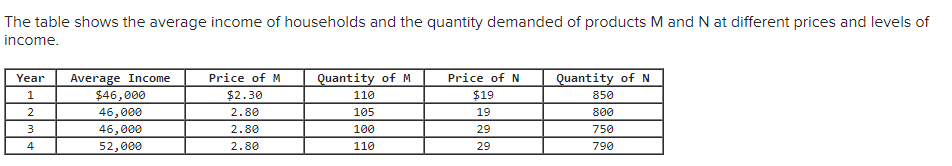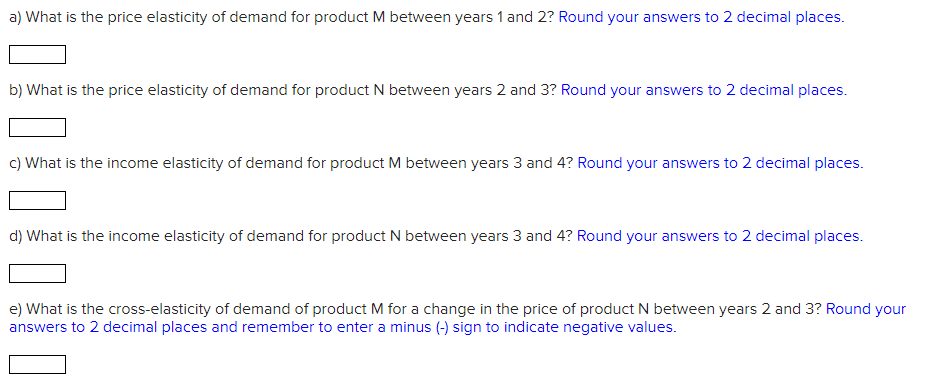# Question The table shows the average income of households and the quantity demanded of products M and N at different prices and levels of income. Year 1 2 3 Average Income \$46,000 46,000 46,000 52,000 Price of M \$2.30 2.80 2.80 2.80 Quantity of M 110 105 100 110 Price of N \$19 19 29 29 Quantity of N 850 800 750 4 790 a) What is the price elasticity of demand for product M between years 1 and 2? Round your answers to 2 decimal places. b) What is the price elasticity of demand for product N between years 2 and 3? Round your answers to 2 decimal places. c) What is the income elasticity of demand for product M between years 3 and 4? Round your answers to 2 decimal places. d) What is the income elasticity of demand for product N between years 3 and 4? Round your answers to 2 decimal places. e) What is the cross-elasticity of demand of product M for a change in the price of product N between years 2 and 3? Round your answers to 2 decimal places and remember to enter a minus (-) sign to indicate negative values.